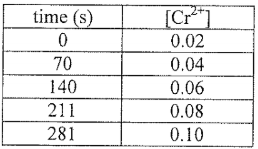# Problem: A student devises an electrochemical cell with the following cell notation:Cr (s) | Cr2+ (aq, 0.02 M) | | Co2+ (aq, 0.9 M) | Co (s)The student monitored the [Cr2+] vs. time. The resultant data is shown in the table below. Determine the current (in amps) produced by this cell during the time period shown. Note: each half-cell has a total volume of 1 L.  Show all work and circle your final answer.Note: This procedure will work for any of the points given above:

###### FREE Expert Solution

For this problem, we have to calculate the current produced by the cell during the time period in the table

• In this case, we will be using 0-70 seconds as a reference to get the current
92% (44 ratings)###### Problem Details

A student devises an electrochemical cell with the following cell notation:

Cr (s) | Cr2+ (aq, 0.02 M) | | Co2+ (aq, 0.9 M) | Co (s)

The student monitored the [Cr2+] vs. time. The resultant data is shown in the table below. Determine the current (in amps) produced by this cell during the time period shown. Note: each half-cell has a total volume of 1 L.  Show all work and circle your final answer.Note: This procedure will work for any of the points given above: